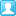##用户名 Email 自动登录 找回密码 密码 立即注册
 搜索

# [其它] Ren'Py中使用反射(reflection)，根据字符串执行模块或类的方法发表于 2021-3-1 11:19:25 | 显示全部楼层 |阅读模式

### 马上注册，结交更多好友，享用更多功能，让你轻松玩转社区。

×

[RenPy] 纯文本查看 复制代码
```init python:
class Calculator:
return a+b
def sub(self, a, b):
return a-b;
def multiply(self, a, b):
return a*b;
def devide(self, a, b):
# 这里除法结果将返回整形，可以根据需要修改返回类型和精度
return a/b

calculator = Calculator()

def calc(a, b, operator):
# 判断对象中是否包含名为“operator”的方法。
# 注意 operator 入参是字符串
if(hasattr(calculator, operator)):
# 反射技术的核心函数
func = getattr(calculator, operator)
store.result = str(func(a, b))
else:
store.result = ""

define a = 3
define b = 2
define operator = ""
default result = ""

screen test():

text "变量a：[a]" xpos 0.4 ypos 0.3
text "变量b：[b]" xpos 0.6 ypos 0.3
text "计算结果：[result]" xpos 0.5 ypos 0.5

hbox:
xpos 0.5
ypos 0.7

textbutton _("相加") action Function(calc, a, b, "add")
textbutton _("相减") action Function(calc, a, b, "sub")
textbutton _("相乘") action Function(calc, a, b, "multiply")
textbutton _("相除") action Function(calc, a, b, "devide")

return

define e = Character("艾琳")

label start:

call screen test```

### 相关帖子

 本版积分规则 回帖后跳转到最后一页

GMT+8, 2023-12-1 12:27 , Processed in 0.037476 second(s), 15 queries , File On.